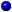HREF="s9node1.html">9.1 Typesetting with TEX  Up: 9 LEARNING LABORATORY ENVIRONEMENT  Next: 9.3 VMarket parameters and

9.2 Programming in JAVA

Slide : [ Java || VMARKET tree - names || JAVA tutorial ]

The numerical schemes submitted from the Java window are automatically inserted in the VMARKET source code (e.g. solution 2.03) and compiled on our server before you can download them for execution locally in your browser. This section introduces a limited number of Java commands that will be useful when you carry out your assignments. More details concerning the VMARKET applet can be found in the program tree, the name index and finally in the program listing in the course environement. For a complete course in Java programming, consult the website from Sun Microsystems.VMARKET = Virtual MARKET applet.

The VMARKET applet is a wrapper to perform a stepwise evolution of functions, i.e. solving time-dependent differential equations using algorithms that can schematically be written as
1. At time=0 use the well known terminal payoff of a contract to initialize functions f0[i],f[i],g[i], the exact definition of which depend on the specific problem.
2. Plot f (black curve), f0 (grey), and g (blue).
3. Use the Java-code submitted in an assignment to calculate the new values for fp after a small time step in terms of present f and sometimes past values fm.
4. Shift the time levels time=time+timeStep and the solution arrays fm=f; f=fp.
5. Goto 2 until finished.
Only the third step can be modified by the user in the Java window; for example, a simple loop
double scale = runData.getParamValue("UserDouble");
for (int i=0; i<=n; i++) {
fp[i]=scale*f[i];
}
executes an artificial evolution, where the next value fp is obtained from a simple scaling of the present value f. Remember that you have to force your browser to reload the applet after each modification in order to prevent your browser from using an older version of the applet that has been temporarily stored in the browser data cache.VMARKET variables.

From the list of run parameters (a Java object called runData), the VMARKET applet first defines local variables (double = 16 digits precision real, int = 9 digits signed integer) using statements
 vanilla call option boolean isCall=ic.equals(vmarket.CALL); vanilla put option boolean isPut =ic.equals(vmarket.PUT); total run time double rTime=runData.getParamValue("RunTime"); drift double mu =runData.getParamValue("Drift"); volatility double sigma=runData.getParamValue("Volatility"); distribution double kappa=runData.getParamValue("LogNkappa"); interest rate double rate =runData.getParamValue("SpotRate"); dividend double divid=runData.getParamValue("Dividend"); exercise price double strke=runData.getParamValue("StrikePrice"); relative barrier double bar =runData.getParamValue("Barrier"); market price of risk double lamb =runData.getParamValue("MktPriceRsk"); mean reversion value double ca =runData.getParamValue("MeanRevTarg"); mean reversion speed double cb =runData.getParamValue("MeanRevVelo"); initial condition double ic0 =runData.getParamValue("Shape0"); lower value x-axis double x0 =runData.getParamValue("MeshLeft"); length of x-axis double len =runData.getParamValue("MeshLength"); number mesh points int n =runData.getParamValueInt("MeshPoints"); number realizations int N =runData.getParamValueInt("Walkers"); time step double step =runData.getParamValue("TimeStep")(); implicit time param double theta=runData.getParamValue("TimeTheta"); tunable quadrature double tune =runData.getParamValue("TuneQuad"); user defined integer int myInt=runData.getParamValueInt("UserInt"); user defined real double myDbl=runData.getParamValue("UserDouble");

The evolution is then computed with the help of predefined arrays containing the solution (an object called solution)
 time in the simulation double time; last index of solution int n = x.length-1; mesh points, intervals double[] x,dx; solution functions double[] f0,f,g; old, present future double[] fm,f,fp; derivatives double[] dfm,df,dfp; current realization double[][] currentState;

Note that in Java (as in C and C++), the index of arrays starts with zero (x) and finishes with an index lower by one element less than its size (x[x.length-1]).Debugging.

Having corrected all the compiler errors does unfortunately not mean that your scheme immediately behaves the way you want... You may have to monitor the value of the quantities you defined, using statements of the form
/* the mistake dividing by zero has been commented out for debugging
double error = fp[n]/0;
*/
System.out.println("Value fp["+i+"] = "+fp[i]);
This example will print the values of the array fp to the Java Console of your browser (with Netscape select Communicator + Tools + Java Console, with Explorer first select Tool + Internet Options + Advanced + Java console enabled and then View + Java Console). From the values that are printed after a single step, it is possible to track down most of the mistakes. Another debuging strategy is to temporarily des-activate a portion of your program, using the \* Java comment delimiters *\ that can span over several lines.Common errors.

Avoid the most common difficulties when you start programming for your assignments
• Every new variable that is not explicitly listed in the variable index) has to be declared; in particular, memory must be allocated for arrays and objects using the command new
int i = 3;                // Declare i as an integer
double[] c;               // Declare c[] array 16 digits nbrs
c = new double[i];        // Memory for c, c, c
BandMatrix  A;            // Declare A as a BandMatrix object
A = new BandMatrix(3,10); // Memory for 3 bands with 10 doubles

• Accessing the element c before the memory has been attributed with a new statement leads to the infamous java.lang.NullPointerException error; using c throws an java.lang.ArrayIndexOutOfBoundsException:3, since the array is accessed outside its valid range 0,1,2.

• In Java, the assigning equal sign is denoted by as single = whereas the comparing equal sign by a double ==
int a = 42;
if (a == 17) System.out.println("a is equal to 17");
if (a != 17) System.out.println("a is not equal to 17");

will print the text "a is not equal to 17" to the Java Console.

SYLLABUS  Previous: 9.1 Typesetting with TEX  Up: 9 LEARNING LABORATORY ENVIRONEMENT  Next: 9.3 VMarket parameters and